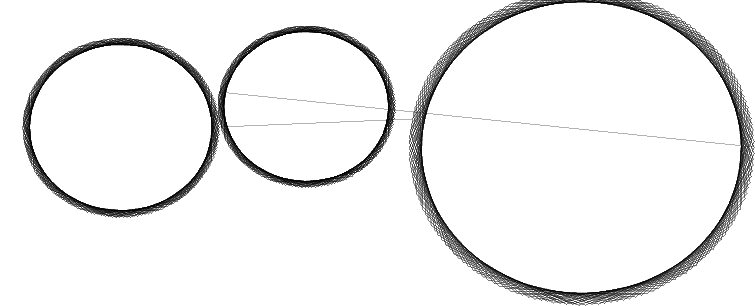# How to handle Datachange signal for circle diagram?

• Hi,
I'm drawing circle diagram using my own inbuilt libraries.
I'm able to draw when i m giving data in diff variable (x,y &r) ,sharing code:

``````double step = (2 * 3.14) / 20;
double cx = 0, cy = 0, r = 1.1563, cx2 = 3.355, cy2 = -0.588, r2 = 2.25, cx3 = -2.419, cy3 = -0.302, r3 = 1.281,
cx4 = -4.484, cy4 = -2.347, r4 = 1.6250
//drawing circle 1-working code
CHPlotCurveData* curvedata1 = new CHPlotCurveData();
CHPlotCurveData* curvedata2 = new CHPlotCurveData();
for (double angle = 0; angle <= 360; angle++)
{
double theta = (angle * 180) / 3.14;
double x = cx + r * cos(theta);
double y = cy + r * sin(theta);
QPointF pt(x, y);
curvedata1->append((pt));
}
for (double angle = 0; angle <= 360; angle++)
{
double theta = (angle * 180) / 3.14;
double x2 = cx2 + r2 * cos(theta);
double y2 = cy2 + r2 * sin(theta);
QPointF pt2(x2, y2);
curvedata2->append((pt2));
}
CHPlotCurve* curve1 = (CHPlotCurve*)pGr->insertCurve("circle1",
CHPlotGraph2D::Line, false );
curve1->setSamples(curvedata1);
CHPlotCurve* curve2 = (CHPlotCurve*)pGr->insertCurve("circle2",
CHPlotGraph2D::Line, false );
curve2->setSamples(curvedata2);
``````

I need to draw circles using table data x,y and radius value from table1(Qtableview) how should i do?

`````` //draw circles using values from table1-not working code
QAbstractItemModel* table1 = ui.tableView->model();
for (int irows = 0, maxI = table1->rowCount(); irows < maxI; ++irows)
{
double x1 = table1->data(table1->index(irows, 1)).toDouble(&ok1);
double y1 = table1->data(table1->index(irows, 2)).toDouble(&ok2);
double r = table1->data(table1->index(irows, 6)).toDouble(&ok3);
for (double angle = 0; angle <= 360; angle++)
{
double theta = (angle * 180) / 3.14;
double zx = x1 + r * cos(theta);
double zy = y1 + r * sin(theta);
QPointF pt(zx, zy);
curvedata1->append((pt));
}
}
CHPlotCurve* curve1 = (CHPlotCurve*)pGr->insertCurve("circle1",
CHPlotGraph2D::Line, false );
curve1->setSamples(curvedata1);
``````

As I'm appending point value so don't understand how to do ?
Thank You

• You do not say what you actually see and why you think it is wrong.

Have you checked the three booleans ok1, ok2, and ok3?
Have you checked the values you receive as x1, x2, and r?
Are you intending to append the coordinates for all circles to the same curvedata1?
Where is curvedata1 being used to draw?

• yes, i checked i am getting value in x,y &r
actually this i didnt understood how i can append my curvedata like there are diff value for rows
yes i used curvedata1 after loop edited code

• You need to plot inside the outer loop (i.e. once per row) and either empty curvedata1, or create a new instance of whatever class that is, in preparation for the next row. (I do not know what library CHPlotCurve etc. belongs to).

• @ChrisW67 inside outer loop plot then also one circle is creating & multiple graph, i want one graph with multiple circles.
I'm using datachange signal with these, as in table data can be change but its creating no. of graphs ..don't understand how to approach..

CHPlotGraph2D - this class to create graph
CHPlotCurveData- this Class hold the data points for the curves
CHPlotCurve-Class to draw the data as a line curve

• Whenever i enter any value in item everytime graph is creating for that how to solve this ?
Circles are coming but actually graph is coming multiple times as bcz im using datachange signal how to overcome with this ?
like if i change data in my table then only single graph can come with multiple circle based on my table entries.

• As i am using datachange signal,Is there other signal i can use or what change i can make in code ?? so that graph with circle come one time, if user enter any value in table then not effect the graph (draw circle when x y r value entered)

• @n-2204 If you add a new curve every time the table model says the data changed, then you will get a lot of curves over time. When anything changes in the table, you need to remove the existing curve containing all the circles and build/add a new one, or replace the data that the existing curve is using to plot. You need to keep a track of the CHPlotCurve object(s) as a member variable to enable removing it from the CHPlotGraph2D object, or replace its data.

• @ChrisW67 Thank you
I have done same thing.
but one issue is like when circle drawing first then next so some lines are coming like we draw circle without removing pen from graph.• This post is deleted!